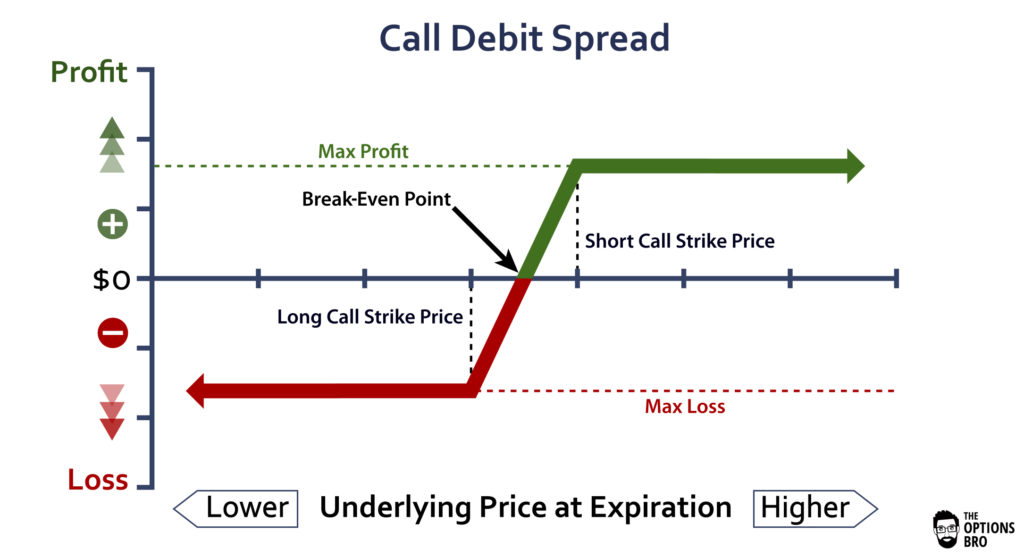21
Mar 21A combination of several options in one trade is called a strategy. Here we discuss a strategy called a call debit spread. The word "debit" in this name means that a trader has to pay for it. The rule of thumb is that if it is a debit (you pay for a strategy), then it is less risky than if it is a credit (you are paid). Let$c(K)$ denote the call price with the strike$K,$ suppressing all other variables that influence the call price.

Assumption. The market values higher events of higher probability. This is true if investors are rational and the market correctly reconciles views of different investors.

We need the following property: if$K_{1} are two strike prices, then for the corresponding call prices (with the same expiration and underlying asset) one has$c(K_{1})>c(K_{2}).$

Proof.  A call price is higher if the probability of it being in the money at expiration is higher. Let$S(T)$ be the stock price at expiration$T.$ Since$T$ is a moment in the future,$S(T)$ is a random variable. For a given strike$K,$ the call is said to be in the money at expiration if$S(T)>K.$ If$K_{1} and$S(T)>K_{2},$ then$S(T)>K_{1}.$ It follows that the set$\{ S(T)>K_{2}\}$ is a subset of the set$\{S(T)>K_{1}\} .$ Hence the probability of the event$\{S(T)>K_{2}\}$ is lower than that of the event$\{S(T)>K_{1}\}$ and$c(K_{1})>c(K_{2}).$

Call debit spread strategy. Select two strikes$K_{1} buy$c(K_{1})$ (take a long position) and sell$c(K_{2})$ (take a short position). You pay$p=c(K_{1})-c(K_{2})>0$ for this.

Our purpose is to derive the payoff for this strategy. We remember that if$S(T)\leq K,$ then the call$c(K)$ expires worthless.

Case$S(T)\leq K_{1}.$ In this case both options expire worthless and the payoff is the initial outlay: payoff$=-p.$

Case$K_{1} Exercising the call$c(K_{1})$ and immediately selling the stock at the market price you gain$S(T)-K_{1}.$ The second option expires worthless. The payoff is: payoff$=S(T)-K_{1}-p.$ (In fact, you are assigned stock and selling it is up to you).

Case$K_{2} Both options are exercised. The gain from$c(K_{1})$ is, as above,$S(T)-K_{1}.$ The holder of the long call$c(K_{2})$ buys from you at price$K_{2}.$ Since your position is short, you have nothing to do but comply. You buy at$S(T)$ and sell at$K_{2}.$ Thus the loss from$-c(K_{2})$ is$K_{2}-S(T).$ The payoff is: payoff$=\left(S(T)-K_{1}\right) +\left( K_{2}-S(T)\right) -p=K_{2}-K_{1}-p.$

Summarizing, we get:

payoff$=\left\{\begin{array}{ll} -p, & S(T)\leq K_{1} \\ S(T)-K_{1}-p, & K_{1}

Normally, the strikes are chosen so that$K_{2}-K_{1}>p.$ From the payoff expression we see then that the maximum profit is$K_{2}-K_{1}-p>0,$ the maximum loss is$-p$ and the breakeven stock price is$S(T)=K_{1}+p.$ This is illustrated in Figure 1, where the stock price at expiration is on the horizontal axis.Figure 1. Payoff for call debit strategy. Source: https://www.optionsbro.com/

Conclusion. For the strategy to be profitable, the price at expiration should satisfy$S(T)\geq K_{1}+p.$ Buying a call debit spread is appropriate when the price is expected to stay in that range.

In comparison with the long call position$c(K_{1}),$ taking at the same time the short call position$-c(K_{2})$ allows one to reduce the initial outlay. This is especially important when the stock volatility is high, resulting in a high call price. In the difference$c(K_{1})-c(K_{2})$ that volatility component partially cancels out.

Remark. There is an important issue of choosing the strikes. Let$S$ denote the stock price now. The payoff expression allows us to rank the next choices in the order of increasing risk: 1)$K_1 (both options are in the money, less risk), 2)$K_1 and 3)$K_1 (both options are out of the money, highest risk).  Also remember that a call debit spread is less expensive than buying$c(K_{1})$ and selling$c(K_{2})$ in two separate transactions.

Exercise. Analyze a call credit spread, in which you sell$c(K_{1})$ and buy$c(K_{2})$.